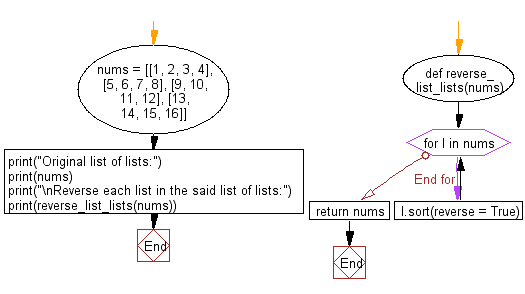﻿ Python: Reverse each list in a given list of lists - w3resource# Python: Reverse each list in a given list of lists

## Python List: Exercise - 129 with Solution

Write a Python program to reverse each list in a given list of lists.

Sample Solution:

Python Code:

``````def reverse_list_lists(nums):
for l in nums:
l.sort(reverse = True)
return nums
nums = [[1, 2, 3, 4], [5, 6, 7, 8], [9, 10, 11, 12], [13, 14, 15, 16]]
print("Original list of lists:")
print(nums)
print("\nReverse each list in the said list of lists:")
print(reverse_list_lists(nums))
```
```

Sample Output:

```Original list of lists:
[[1, 2, 3, 4], [5, 6, 7, 8], [9, 10, 11, 12], [13, 14, 15, 16]]

Reverse each list in the said list of lists:
[[4, 3, 2, 1], [8, 7, 6, 5], [12, 11, 10, 9], [16, 15, 14, 13]]
```

Flowchart:## Visualize Python code execution:

The following tool visualize what the computer is doing step-by-step as it executes the said program:

Python Code Editor:

Have another way to solve this solution? Contribute your code (and comments) through Disqus.

What is the difficulty level of this exercise?

Test your Python skills with w3resource's quiz

﻿

## Python: Tips of the Day

Floor Division:

When we speak of division we normally mean (/) float division operator, this will give a precise result in float format with decimals.

For a rounded integer result there is (//) floor division operator in Python. Floor division will only give integer results that are round numbers.

```print(1000 // 300)
print(1000 / 300)```

Output:

```3
3.3333333333333335```